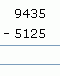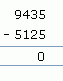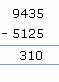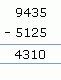Name: ___________________Date:___________________

 Email us to get an instant 20% discount on highly effective K-12 Math & English kwizNET Programs!

### Grade 3 - Mathematics Subtraction - 4 Digits

Method:

1. Start subtracting from the ones place.
2. If the subtrahend is lesser than minuend then we borrow 1 from tens place.
3. If the subtrahend is lesser than minuend in tens place then we borrow 1 from hundreds place.
4. If the subtrahend is lesser than minuend in hundreds place then we borrow 1 from thousands place.

Subtraction without regrouping:

Example 1:
 Question Step 1 Step 2 Step 3 Step 41. Start from ones place 5 minus 5 is 0.
2. In the tens place, 3 minus 2 is 1.
3. In the hundreds place 4 minus 1 is 3.
4. In thousands place, 9 minus 5 is 4.

Example 2:1. Start from ones place 8 minus 6 is 2.
2. In the tens place, 9 minus 9 is 0.
3. In the hundreds place 9 minus 8 is 1.
4. In thousands place, 4 minus 2 is 2.

Subtraction with regrouping:

Example:1. Start from ones place 7 minus 2 is 5.
2. In the tens place 3 is less than 8, so 1 is borrowed from 0 which has borrowed 1 from 3. So the result in tens place is 13 minus 8 which is 5.
3. In the hundreds place 0 becomes 9, the result is 3.
4. In thousands place, 3 becomes 2 and the result is 1.

Directions: Subtract the following.
 Question 1: 9381 - 6128

 Question 2: 3497 - 2433

 Question 3: 4628 - 3264

 Question 4: 1757 - 1526

 Question 5: 8232 - 7634

 Question 6: 8542 - 2810

 Question 7: 654 - 53

 Question 8: 7870 - 7452

 Question 9: 8207 - 1939

 Question 10: 1083 - 253

 Question 11: 7782 - 5310

 Question 12: 6604 - 3235

 Question 13: 7787 - 1014

 Question 14: 9244 - 612

 Question 15: 5858 - 1481

 Question 16: 7809 - 1986

 Question 17: 1306 - 862

 Question 18: 4420 - 4126

 Question 19: 5946 - 5934

Question 20: This question is available to subscribers only!

Question 21: This question is available to subscribers only!

Question 22: This question is available to subscribers only!

Question 23: This question is available to subscribers only!

Question 24: This question is available to subscribers only!